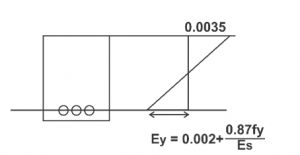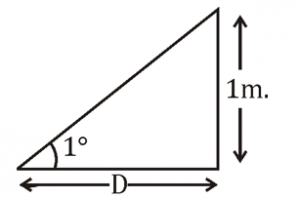Engineering Jobs   »   Remove term: DFCCIL Civil engineering quiz...

# DFCCIL’21 CE: Daily Practice Quiz. 21-July-2021

Quiz: Civil Engineering
Exam: DFCCIL-JE
Topic: MISCELLANEOUS

Each question carries 1 mark
Negative marking: 1/4 mark
Time: 8 Minutes

Q1. Maximum strain in an extreme fiber in concrete and in the tension reinforcement (Fe-415 Grade and E_s=200 kN/mm²) in a balanced section at limit state of flexure are respectively.
(a) 0.0035 and 0.0038
(b) 0.002 and 0.0018
(c) 0.0035 and 0.0041
(d) 0.002 and 0.0031

Q2. According to IS 456, nominal mix concrete can be used up to which of the following grade
(a) 10
(b) 15
(c) 20
(d) 25

Q3. A gradient of 1° represents a rise or fall of 1m in a horizontal distance of
(a) 57.3 m
(b) 44.7 m
(c) 63.3 m
(d) 68.7 m

Q4. Assuming the safe stopping sight distance to be 80m on a flat highway section and with a setback distance of 10m. what would be the radius of the negotiable horizontal curve?
(a) 800 m
(b) 160 m
(c) 80 m
(d) 70 m

Q5. Which of the following curves can be used to avoid overturning of vehicles on a curve?
(a) Reverse
(b) Transition
(c) Vertical
(d) Compound

Q6. Highway geometrics are designed for:
(a) 50th percentile speed
(b) 85th percentile speed
(c) 95th percentile speed
(d) 98th percentile speed

SOLUTION

S1. Ans.(a)
Sol.Maximum strain in extreme fibre of concrete
= 0.0035
Maximum strain in tension reinforcement =
= 0.002+ (0.87×415)/(200×10^3 )
= 0.0038

S2. Ans.(c)
Sol. Nominal mix design used up to M20 Concrete.
M10→1:3∶6
M15→1:2:4
M20→1:1.5:3
→ For M25 onward’s we use design mix.

S3. Ans.(a)Sol. Let horizontal distance = D
Slope = 1°
Rise/fall = 1m

Tan 1° = 1/D
D = 1/(Tan 1° )
= 1/0.017455
D = 57.3 m

S4. Ans.(c)
Sol. Given, SSD = 80 m.
Set back distance(M) = 10m.
Set back distance (M) = S²/8R (Approx. relation)
10 = (80)^2/8R
R = (80×80)/(10×8)
= 80 m.

S5. Ans.(b)
Sol. Overturning of vehicles on a carve can be avoided by using transition curve. transition curve is provided between straight and circular curve.

S6. Ans.(d)
Sol. Highway Geometric are designed for 98th percentile speed.

Sharing is caring!